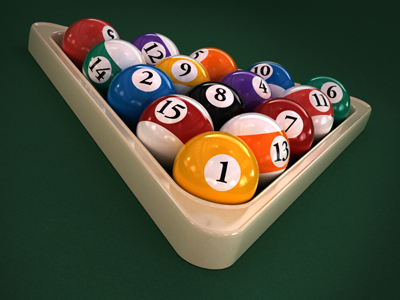This triangle used in the game of pool is an equilateral triangle.

# Level 3-4 Shapes - Angles

Shapes are a key part of KS3 Maths. One feature found in shapes is angles. This quiz will find out whether you've got the angle on your angles!

An angle is a measure of turning or rotation. Angles are measured in degrees, which are shown by this sign o. There are 360o in a circle and 180o in a half-circle or straight line. Right angles (the corners on squares) have 90o and all of the angles in a triangle add up to 180o. Angles less than 90o are called acute angles, those between 90o and 180o are obtuse and those over 180o are reflex. There are many more facts to learn about angles. This quiz will help you get to grips with the subject.

Take your time over the following questions. Read each one carefully and make sure you understand it before you choose which answer is correct. And don't forget the helpful comments which appear after you've answered a question. These can help to clarify anything which you didn't understand. Good luck!.

1.
An angle that is between 0o and 90o is a(n) .......
acute angle
obtuse angle
reflex angle
right angle
Think of obtuse angles as 'blunt' and acute angles as 'sharp'
2.
The internal angles of a regular hexagon add up to 720o. How many degrees is each one?
60o
90o
120o
150o
There are six internal angles in a hexagon. A regular hexagon has six equal angles
3.
Each internal angle of a regular pentagon is 108o. What is the total number of internal degrees?
180o
360o
540o
720o
Pentagons have 5 internal angles so just multiply 108o x 5
4.
The three interior angles in a triangle add up to what?
90o
180o
270o
360o
An equilateral triangle has 3 interior angles each measuring 60 degrees each (3 x 60 = 180)
5.
An angle that is 90o is a(n) .......
acute angle
obtuse angle
reflex angle
right angle
These are found in squares and other rectangles
6.
An angle that is between 90o and 180o is a(n) .......
acute angle
obtuse angle
reflex angle
right angle
Think of obtuse angles as 'blunt' and acute angles as 'sharp'
7.
Two interior angles in a triangle are 35o and 55o. What is the 3rd angle?
75o
85o
90o
95o
Take 35 and 55 from 180 to find the answer
8.
An angle that is between 180o and 360o is a(n) .......
acute angle
obtuse angle
reflex angle
right angle
Every internal angle has a corresponding reflex angle. Together they add up to 360o
9.
A circle is divided into a number of parts or degrees. How many are there?
90o
180o
270o
360o
The four interior angles of a quadrilateral also add up to 360o
10.
Angles on a straight line add up to what?
90o
180o
270o
360o
A straight line is half a circle